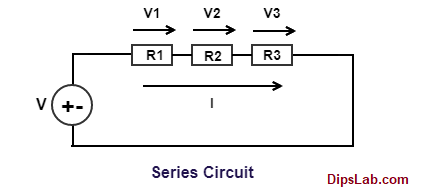# Series and Parallel Circuit Calculator

### Equivalent Series and Parallel Circuit Calculator

Here, we are studying about the equivalent or total series circuit and parallel circuit connection calculation.

Firstly, you should know about  basic concept such as the series and parallel circuit connection with connected different elements.

In an earlier tutorial, I have explained about the Series Circuit and Parallel Circuit with an example.

#### 1. Series Circuit Calculator-

In a series circuit connection, the number of electrical elements or components are connected in series or sequential form.

For example, the given circuit is said to be series circuit, when electronics components (such as resistance R1, R2 and R3) are connected in a single path with connected voltage source (Vs).When voltage source is given to a circuit, the same current is flowing (I). But, different (or drop) voltage (V1, V2, and V3) occurred across all the serial connected resistance.

The sum of voltage drops in individual series connected resistances is equal to the applied voltage (i..e V= V1+V2+V3).

Let’s check, here some important formulas for different connected components in series circuit.

For the calculation of Current in Series:

`Total Current, (Ieq) = (I1= I2 = I3 = I4 = 15 = ......= In)         (Unit- Ampere)`
Note: The same current flows or occur at the different connected components in series circuit.

For the calculation of voltage in Series:

`Total Current, (Veq) = (V1+ V2 + V3 + V4 = V5 = ......= Vn)         (Unit- Volt)`
For the calculation of Resistance in Series:

`Total Resistance, (Req) = (R1+ R2 + R3 + R4 + R5 +......+ Rn)         (Unit- Ohm)`
For the calculation of Conductance in Series:

`Total Conductance, (Geq) = [1 / ((1/G1) + (1/G2) + (1/G3) + (1/G4) + (1/G5) +......+ (1/Gn))]          (Unit- Siemens)`
For the calculation of Capacitance in Series:

`Total Capacitance, (Ceq) = [1 / ((1/C1) + (1/C2) + (1/C3) + (1/C4) + (1/C5) +......+ (1/Cn))]       (Unit- Farad)`
For the calculation of Inductance in Series:

`Total Inductance, (Leq) = (L1 + L2 + L3 + L4 + L5......+ Ln)         (Unit- Henry)`

This is the simple description about the series circuit with the different formulas.

#### 2. Parallel Circuit Calculator:

In the parallel circuit connection, the number of electrical elements or components are connected in parallel form.

For example, when electronics components (such as resistance R1, R2 and R3) are connected in a parallel branch with connected voltage source (Vs).When voltage source is given to a circuit, the same current is flowing (I). But, different (or drop) voltage (V1, V2, and V3) occurred across all the parallel connected resistance.

The sum of voltage drops in individual parallel connected resistances is equal to the applied voltage (i..e V= V1+V2+V3).

Let’s check, here some important formulas for different connected components in parallel circuit.

For the calculation of Current in Parallel:

`Total Current, (Ieq) = (I1 + I2 + I3 + I4 + 15 + ......+ In)         (Unit- Ampere) `
For the calculation of Voltage in Parallel:

`Total Voltage, (Veq) = (V1 = V2 = V3 = V4 = V5 =......+ Vn)         (Unit- Volt)`
Note: The same voltage or potential difference occur at the different connected components.

For the calculation of Resistance in Parallel:

`Total Resistance, (Req) = [1/((1/R1) + (1/R2) + (1/R3) + (1/R4) + (1/R5) +......+ (1/Rn))]         (Unit- Ohm)`

For the calculation of Conductance in Series:

`Total Conductance, (Geq) = (G1 + G2 + G3 + G4 + G5 +......+ Gn)          (Unit- Siemens)`

For the calculation of Capacitance in Series:

`Total Capacitance, (Ceq) =  (C1 + C2 + C3 + C4 + C5 +......+ Cn)   (Unit- Farad)`
For the calculation of Inductance in Series:
`Total Inductance, (Leq) = [1 / ((1/L1) + (1/L2) + (1/L3) + (1/L4) + (1/L5) +......+ (1/Ln))]         (Unit- Henry)`
This is the simple description about the parallel circuit with the different formulas.